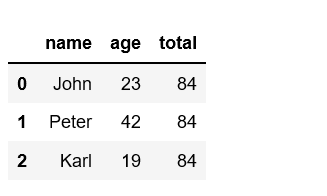# Python | Pandas Series.sum()

Python is a great language for doing data analysis, primarily because of the fantastic ecosystem of data-centric python packages. Pandas is one of those packages and makes importing and analyzing data much easier.

Pandas `Series.sum()` method is used to get the sum of the values for the requested axis.

Syntax: Series.sum(axis=None, skipna=None, level=None, numeric_only=None, min_count=0)

Parameters:
axis : {index (0)}
skipna[boolean, default True] : Exclude NA/null values. If an entire row/column is NA, the result will be NA
level[int or level name, default None] : If the axis is a MultiIndex (hierarchical), count along a particular level, collapsing into a scalar.
numeric_only[boolean, default None] : Include only float, int, boolean data. If None, will attempt to use everything, then use only numeric data

Returns: Returns the sum of the values for the requested axis

Code #1: By default, the sum of an empty or all-NA Series is 0.

 `# importing pandas module  ` `import` `pandas as pd  ` ` `  `# min_count = 0 is the default ` `pd.Series([]).``sum``() ` ` `  `# When passed  min_count = 1, ` `# sum of an empty series will be NaN ` `pd.Series([]).``sum``(min_count ``=` `1``) `

Output:

```0.0
nan```

Code #2:

 `# importing pandas module  ` `import` `pandas as pd  ` `   `  `# making data frame csv at url   ` `data ``=` `pd.read_csv(``"https://media.geeksforgeeks.org/wp-content/uploads/nba.csv"``)  ` ` `  `# sum of all salary ` `val ``=` `data[``'Salary'``].``sum``() ` ` `  `val `

Output:

`2159837111.0`

Code #3:

 `# importing pandas module  ` `import` `pandas as pd  ` `   `  `# making a dict of list ` `data ``=` `{``'name'``: [``'John'``, ``'Peter'``, ``'Karl'``], ` `        ``'age'` `: [``23``, ``42``, ``19``]} ` ` `  `val ``=` `pd.DataFrame(data) ` ` `  `# sum of all salary ` `val[``'total'``] ``=` `val[``'age'``].``sum``() ` ` `  `val `

Output:My Personal Notes arrow_drop_upCheck out this Author's contributed articles.

If you like GeeksforGeeks and would like to contribute, you can also write an article using contribute.geeksforgeeks.org or mail your article to contribute@geeksforgeeks.org. See your article appearing on the GeeksforGeeks main page and help other Geeks.

Please Improve this article if you find anything incorrect by clicking on the "Improve Article" button below.

Article Tags :

Be the First to upvote.

Please write to us at contribute@geeksforgeeks.org to report any issue with the above content.